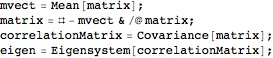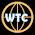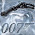Wednesday, August 17, 2011

Build the vectors and Feature Reduction

Part I: building the vectors
In the former post we have created a features set for the class GOLD, now we show how use this features set to build the vectors to feed the svm.
The Features set is the fingerprint that characterizes the document class.
To build the vectors, you have to follow the same parsing and filtering process used to extract the features.
As described in the previous post,  from the original file, you have to follow the below steps:
• Split the words of the file;
• Remove the words having Length <= 2;
• Remove the stopWords
• Take the "root" of the word (e.g. Large from Larger)
On the filtered file just obtained, you can build every sort of statistic to associate to each word of the filtered file a score.
For our purpose the simple frequency could be enough.
The last step is to associate to each word of the filtered file the score just calculated with the score associated to each word of the features set.
This step is illustrated in the below illustration:"FT score" stands for Feature set score, < . , . > stands for a linear operator. Notice that a vector assumes 0 value when the filtered file doesn't contain occurrences for the specific feature. The grey box is the final Real Number vector.
As you can see the vector has the same dimension of the Feature Set, in this case (built using TF-DF) it has dimension equal closed to 1000.
In a enterprise environment it could be a bottleneck in terms of computational capabilities, this due to the strict relation between vector dimension and time complexity to classify.

Part II: reducing the dimension
How can we reduce the dimension of the problem to speed up the entire classification process?
There are different methods,  the first I would show is the Principal Component Analysis (PCA).
As usual, I'm not going to enter in technical and theoretical issues: there are thousands websites, tutorial and paper  where you can find every sort of details on that.
Just to have an overview, PCA provides the most important linear components in a set of data. It is based on the concept of eigenvectors and eigenvalues : M.x = λ x where λ is the eigenvalue and x is the eigenvector.
PCA has two great features:
1. it is completely unparameterized;
2. it is extremely easy to implement;
In Mathematica the implementation takes no more than 4-5 rows code:the variable matrix is the dataset having whatever Dimension

Basically you standardize the data (to have mean = 0),  you compute the covariance matrix, and on that you extract the eigenvalues and eigenvectors.
The position of eigenvectors having higher eigenvalues are the the component most relevant: the components relevant are the features most important to classify the documents with a minimum loss of accuracy!
Just to understand the role played by eigenvectors consider  the following multivariate gaussian data set:Data set gaussian distributed

The principal components are well represented by the red vectors:Principal Components for the above data set
Nevertheless, PCA is not useful in data having different density, and before to discard components (features) from your data set, I suggest to analyze the density!

What happens in our classes?
I computed the PCA for the GOLD class, but the question  now is "What is the minimum threshold to discriminate the important components against the negligible features?
...Sometimes a simple "logPlot" helps the analyst:The blu arrow represent the discontinuity point for the eigenvalues
As shown above, around at the component number 82, there is a discontinuity: after this point the magnitude of the eigenvalue is negligible. Here you are the threshold!
Remark
The vectors have been built using the sorted Feature Set list (see the previous post).
and the criteria to sort was based on the TF- DF (or TF IDF, or ...): from the most important from TF DF prospective to the less important.
The graph above provides us a sort of countercheck of the goodness of the criteria chosen to select the features, because there is a perfect correspondence among the weight assigned with TF DF and the eigenvalues (notice the monotonicity of the graph)!
So for the GOLD class, we will consider only the first 82 components.
As you can see we used PCA in two different ways:
1) to countercheck our features extraction method
2) to reduce the complexity of the problem from 1000 to 82.

In the next post, we will see another algorithm of feature reduction: C4.5.
...We are now introducing many different approaches to play with the data, in the next post I'll show you the graph of experiments we are doing to keep track of every results and to chose the best strategy.
as usual: stay tuned
cristian

1.That is a great practical.
2.3.4.5.6.7.